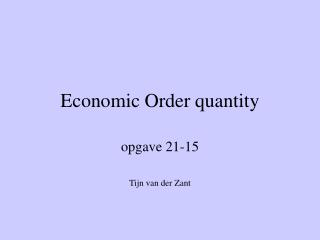DownloadDownload PresentationEconomic Order quantity

# Economic Order quantity

Télécharger la présentation## Economic Order quantity

- - - - - - - - - - - - - - - - - - - - - - - - - - - E N D - - - - - - - - - - - - - - - - - - - - - - - - - - -
##### Presentation Transcript

1. Economic Order quantity opgave 21-15 Tijn van der Zant

2. How to calculate EOQ • EOQ = √(2DP/C) • Where: • D = Demand in units for a period • P = Relevant ordering costs per purchase order • C = Relevant carrying costs of 1 unit in stock for the same period used in D

3. Problem: Keep-Kool company • It buys 12000 units CU29 a ₤50 per unit • 12% annual ROI (return on investment) • Carrying cost per unit per year: ₤2 • Relevant costs per purchase order: ₤120

4. Calculate EOQ for CU29 • D = 12000 • P = 120 • C = 2 • EOQ = √(2*12000*120/2) = 1200

5. Total annual relenant costs (TRC) • Total annual relevant cost (TRC) = DP/Q+QC/2 • Where Q = quantity and in this example I assume it’s the same as EOQ (though that’s not necessary

6. Calculate total ordering and carrying costs using EOQ • DP/Q+QC/2 = 12000*120/1200+1200*2/2 • = 12000 * 1/10 + 1200 • = 1200 + 1200 • = ₤ 2400

7. Reorder Point for CU29 • Reorder point = (number of units sold per unit of time) * (purchase-order lead time) • 12000 units per year = 300 units a week • Lead time = 2 weeks • Reorder point = 300 * 2 = 600 units